THE MoTIS VIRTUAL THEORETICAL AERODYNAMICS DEPARTMENT

WING SECTIONS

WS1.

The basis of all aerodynamics departments is to produce data in an attempt to define the realistic possibility of producing and designing  an efficient and effective aircraft that can function in the area or category in which it was design for. No aircraft on earth operating in a gravitational field can enter the fluid (atmosphere) with out the use of some lift device or pressure differentiation producing device wing, lifting body etc. and use atmospheric physics to exceed equilibrium( thrust and lift over coming weight and drag) and take off into that atmospheric fluid.. Wings are like aerodynamic batteries designed to generate Flow Voltage (Flow Voltage conceptual definition as coined by MoTIS VJD- i.e. not a real world aviation term in current use) difference between fluids at high speeds and fluids at low speeds. Wing Sections or just wings are the basics tools that birds and man uses to affect the nature of a fluid at a uniform speed approaching the wing having a definite shape and volume. We will assume that all are aware of Bernoulli's principle which in its simplified form is a principle related to the inverse relationship of a fluid in motions (non static) fluids velocity and its pressure. In basic the faster the fluid flows the greater the internal pressure of the fluid decreases to some maximum value. Wings in affect are all about using the energy in fluid flow physics to produce what aerodynamics professionals call LIFT! Now we all know that in order for an aircraft to become air born it must use thrust and lift to over come weight and gravity but what we also need to know or should desire to know is what is the mathematical theory to flight and the principles of lift as related to fluid flow or fluid dynamics. This may indeed sound strange but it would do us well to see the aircraft in general as a fish of sorts instead of a flying object from the perspective that a gas flowing is in fact behaving much like a liquid flowing. Our goal at the MoTIS theoretical department is not to use words to ramble on about the principles of flight but to use graphics and animations to build a very detailed picture of flight from a truly theoretical perspective.

So let us start by stating that matter in a gravitational field has weight (W=Mg) , where W = weight and M = mass or matter , and g = the gravitational acceleration unit itself.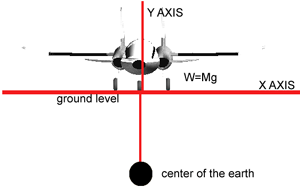Copyrighted (C) 2004 by MoTIS INDUSTRIES.

FIG. WS.1A

 KEY TOPIC EQUATION W=MG

All airplanes have Weight as the wings, engine, and people sitting in the aircraft all add to the ∑ or sum of the total weight of the aircraft under any given circumstance. Theoretically an aircraft with a certain Weight has to over come this weight in order to fly into the sky. As a mater of fact the whole goal of the wing is to provide enough LIFT to allow the weight of the aircraft to be overcome! In order for the wing section of an aircraft do o its job its has to have a certain potential or lifting power vale in order to lift up a certain weight. In other words the wing must be designed in order to achieve a certain task or lifting goal so to speak.

As the gravitational acceleration on an object doesn't change when you add more mass to an aircraft on the ground the added mass causes the weight to increase. Ex.1- Adding mass increases Weight

Now as most people hate the idea of math it is the central foundation of all the sciences and with out it there would be no aircraft in the shies at all! So we mus began to understand some basic math equations that will be needed for our journey into wing sections.

The very first major concept that we all learn in Algebra is the concept of the line equation in what we call slope intercept form.

 KEY TOPIC EQUATION Y=MX+B, SLOPE INTERCEPT FORM

Y=MX+B, AND M=dY/dX, where d=∆ and ∆= Final -Initial of all Y and X  values taken from the Y and X input and output values.

M=dY/dX and M=SLOPE.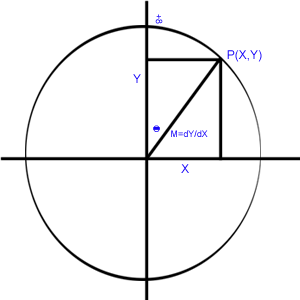Copyrighted (C) 2004 by MoTIS INDUSTRIES.

As the direction of the relative wind of the aircraft creates the direction of the dynamic fluid flow , and the LIFT vector acts perpendicular to the direction of the relative wind, then the mathematical expression of the linear equation can in act be used to express the theoretical dimensions of lift and the dynamic flow of the air acting as a fluid across the wing section!

As we are all aware that the LIFT equation : L=CL x(1/2density)V Squared x S, we will simplify and elucidate the LIFT equation into slope intercept form reducing the core of the equation into an air FLOW / LIFT translation equation setting Bernoulli's principle at a maximum concentration alone the wing that we will call the center of pressure, meaning that the airflow patterns across the wing causes some point on the wing in laminar airflow to have the greatest velocity of airflow and there fore the greatest reduction in internal fluid pressure and becomes the greatest area of lift along the wing and by making lift a function of Flow Voltage (which we will explain more on theoretically later)  as the independent variable and BA= Bernoulli's principle values at a maximum concentrated point where lift perpendicular as a force along the wing Force (F=MA IN A VERTICAL  DIRECTION) out puts. ┴ =PERPENDICULAR TO.

Y=MX+B = LIFT= BA ┴(FV) + B, where B is the maximum value that BA┴ can achieve before it reaches Cl MAX in the general lift equation. As L=∫(FV) d( BA┴), and BA┴=MAX AT CL

M= L/∑ FV, SINCE M=dY/dX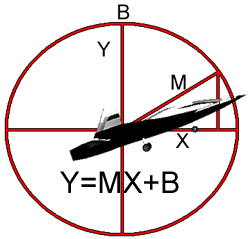Copyrighted (C) 2004 by MoTIS INDUSTRIES.

Ex.2- Speed is to decreasing internal fluid flow pressure and increasing LIFT, as diffusion is too concentration gradients flow from high concentration regions into low concentration regions in an attempt to establish static or dynamic equilibrium depending on the internal energy of the system!!

As the LIFT of an aircraft can only be generated as of the result of having a thrust applied to the mass of the aircraft it is fair to say that the Flow dynamics produced by the aircrafts power plant provides the continuity equation that allows for a +positive FV value and a +positive FV value on an wing with a fixed surface area generates lift in proportion to the angle of attack and the maximum amount of potential pressure difference between the FV velocity difference over and under the wing! Therefore high FV generally means high potential energy differences between the top and bottom of the airfoil and this directly leads to increases and decreases in lift along the airfoil in correlation to changes in V Squared or airspeed from the general lift equation.

 KEY TOPIC EQUATION LIFT=CL x (1/2air density) x (V squared)  x  S, LIFT EQUATION

In the general lift equation the only thing that a pilot can alter on any giving day of flying is the speed of the aircraft and the S or surface area of the wing surface by directly altering the chord of the wing with flaps and control surfaces!

Our fundamental goal is to relate the  Flow Voltage  to the concept of perpendicular LIFT and in all MoTIS designed aircraft the first thing that we concentrate on after establishing the Weight equations, so as to use a reverse integration process for designing our wing dimensions and there are numerous factors to determining just  what types of flow dynamics or thrust ratings  we need to generate the needed amounts of LIFT in order to combat the total Weight of the airplane. The airfoil shape being the biggest contributing to controlling Bernoulli's principle in the wing design process.  As the CL is a design factor designed into the wing section and the air density changes with the weather (i.e man have very little control over these variables with respect to changing the density part of the general lift equation)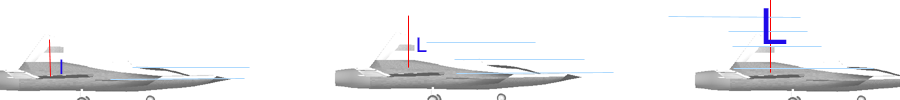Copyrighted (C) 2004 by MoTIS INDUSTRIES.

So as we increase the speed of the aircraft across the wing on a ground takeoff run or wind during  tunnel tests we in fact increase the air flow voltage (FV) over and under the wing that generates the potential pressure difference over and under the wing directly due to the airfoil shape , and this shape is the direct cause of Bernoulli's principle in positive (FV) and the increase in velocity leading to perpendicular translated lift in the form of vertical force (F=M x A)!!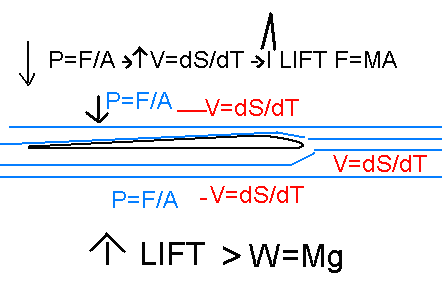Copyrighted (C) 2004 by MoTIS INDUSTRIES.

As the lift equation is complex in its calculative nature we will simplify for the moment what is actual taking place over and under the wings surface.

Copyrighted (C) 2004-2008 by MoTIS INDUSTRIES.

LESSON ONE

Written by MoTIS INDUSTRIES staff writer

NOTE: This section of our website is dedicated to real world aviation agendas and it is expected that all viewers have a basic understanding of calculus and technical mathematics to understand the bases of the equations and data to be added to this site via our student design staff and MoTIS internal staff contributions.